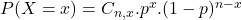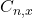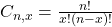## Determine which of the given procedures describe a binomial distribution. Select one: A. Record the number of ear piercings in a group of 30

Question

Determine which of the given procedures describe a binomial distribution. Select one: A. Record the number of ear piercings in a group of 30 randomly selected college students. B. Observing that five out of the next ten customers at a hotdog stand order hot peppers given that the probability of ordering hot peppers is 0.18. C. Surveying customers leaving a hardware store until a customer responds that he or she spent more than fifty dollars.

in progress 0
6 months 2021-07-23T09:23:21+00:00 1 Answers 15 views 0

## Answers ( )

B. Observing that five out of the next ten customers at a hotdog stand order hot peppers given that the probability of ordering hot peppers is 0.18.

Step-by-step explanation:

Binomial probability distribution

The binomial probability is the probability of exactly x successes on n repeated trials, and X can only have two outcomes.In whichis the number of different combinations of x objects from a set of n elements, given by the following formula.And p is the probability of X happening.

Option A:

No, since it is not the probability, or percentage of students that have ear pircings, it is the number, which can assume values larger than 1.

Option B:

Yes, since it is a probability, each customer is independent of other customers, and in each trial, there are only two possible outcomes. This is the correct answer.

Option C:

No, this is called inverse binomial, as we want to find the number of customers until one does something, not the probability of a number of customers doing something.